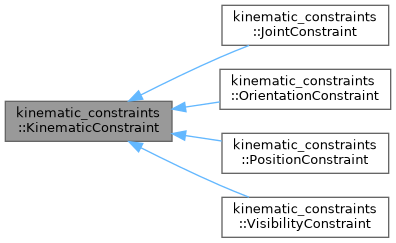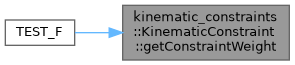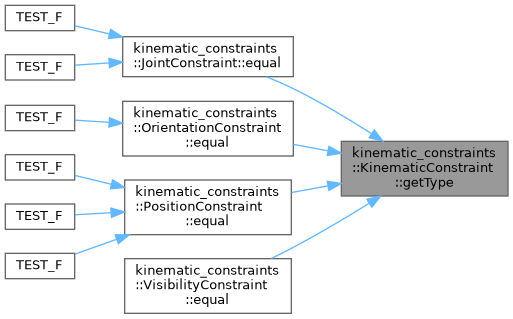moveit2 The MoveIt Motion Planning Framework for ROS 2.
kinematic_constraints::KinematicConstraint Class Referenceabstract

Base class for representing a kinematic constraint. More...

`#include <kinematic_constraint.h>`

Inheritance diagram for kinematic_constraints::KinematicConstraint:[legend]

## Public Types

enum  ConstraintType {
UNKNOWN_CONSTRAINT , JOINT_CONSTRAINT , POSITION_CONSTRAINT , ORIENTATION_CONSTRAINT ,
VISIBILITY_CONSTRAINT
}
Enum for representing a constraint. More...

## Public Member Functions

KinematicConstraint (const moveit::core::RobotModelConstPtr &model)
Constructor. More...

virtual ~KinematicConstraint ()

virtual void clear ()=0
Clear the stored constraint. More...

virtual ConstraintEvaluationResult decide (const moveit::core::RobotState &state, bool verbose=false) const =0
Decide whether the constraint is satisfied in the indicated state. More...

virtual bool enabled () const =0
This function returns true if this constraint is configured and able to decide whether states do meet the constraint or not. If this function returns false it means that decide() will always return true – there is no constraint to be checked. More...

virtual bool equal (const KinematicConstraint &other, double margin) const =0
Check if two constraints are the same. This means that the types are the same, the subject of the constraint is the same, and all values associated with the constraint are within a margin. The other constraint must also be enabled. More...

ConstraintType getType () const
Get the type of constraint. More...

virtual void print (std::ostream &=std::cout) const
Print the constraint data. More...

double getConstraintWeight () const
The weight of a constraint is a multiplicative factor associated to the distance computed by the decide() function. More...

const moveit::core::RobotModelConstPtr & getRobotModel () const

## Protected Attributes

ConstraintType type_
The type of the constraint. More...

moveit::core::RobotModelConstPtr robot_model_
The kinematic model associated with this constraint. More...

double constraint_weight_
The weight of a constraint is a multiplicative factor associated to the distance computed by the decide() function
More...

## Detailed Description

Base class for representing a kinematic constraint.

Definition at line 77 of file kinematic_constraint.h.

## ◆ ConstraintType

Enum for representing a constraint.

Enumerator
UNKNOWN_CONSTRAINT
JOINT_CONSTRAINT
POSITION_CONSTRAINT
ORIENTATION_CONSTRAINT
VISIBILITY_CONSTRAINT

Definition at line 81 of file kinematic_constraint.h.

## ◆ KinematicConstraint()

 kinematic_constraints::KinematicConstraint::KinematicConstraint ( const moveit::core::RobotModelConstPtr & model )

Constructor.

Parameters
 [in] model The kinematic model used for constraint evaluation

Definition at line 127 of file kinematic_constraint.cpp.

## ◆ ~KinematicConstraint()

 kinematic_constraints::KinematicConstraint::~KinematicConstraint ( )
virtualdefault

## ◆ clear()

 virtual void kinematic_constraints::KinematicConstraint::clear ( )
pure virtual

Clear the stored constraint.

## ◆ decide()

 virtual ConstraintEvaluationResult kinematic_constraints::KinematicConstraint::decide ( const moveit::core::RobotState & state, bool verbose = `false` ) const
pure virtual

Decide whether the constraint is satisfied in the indicated state.

Parameters
 [in] state The kinematic state used for evaluation [in] verbose Whether or not to print output
Returns

## ◆ enabled()

 virtual bool kinematic_constraints::KinematicConstraint::enabled ( ) const
pure virtual

This function returns true if this constraint is configured and able to decide whether states do meet the constraint or not. If this function returns false it means that decide() will always return true – there is no constraint to be checked.

## ◆ equal()

 virtual bool kinematic_constraints::KinematicConstraint::equal ( const KinematicConstraint & other, double margin ) const
pure virtual

Check if two constraints are the same. This means that the types are the same, the subject of the constraint is the same, and all values associated with the constraint are within a margin. The other constraint must also be enabled.

Parameters
 [in] other The other constraint to test [in] margin The margin to apply to all values associated with constraint
Returns
True if equal, otherwise false

## ◆ getConstraintWeight()

 double kinematic_constraints::KinematicConstraint::getConstraintWeight ( ) const
inline

The weight of a constraint is a multiplicative factor associated to the distance computed by the decide() function.

Returns
The constraint weight

Definition at line 158 of file kinematic_constraint.h.

Here is the caller graph for this function:## ◆ getRobotModel()

 const moveit::core::RobotModelConstPtr& kinematic_constraints::KinematicConstraint::getRobotModel ( ) const
inline
Returns
The kinematic model associated with this constraint

Definition at line 169 of file kinematic_constraint.h.

## ◆ getType()

 ConstraintType kinematic_constraints::KinematicConstraint::getType ( ) const
inline

Get the type of constraint.

Returns
The constraint type

Definition at line 137 of file kinematic_constraint.h.

Here is the caller graph for this function:## ◆ print()

 virtual void kinematic_constraints::KinematicConstraint::print ( std::ostream & = `std::cout` ) const
inlinevirtual

Print the constraint data.

Parameters
 [in] out The file descriptor for printing

Definition at line 147 of file kinematic_constraint.h.

## ◆ constraint_weight_

 double kinematic_constraints::KinematicConstraint::constraint_weight_
protected

The weight of a constraint is a multiplicative factor associated to the distance computed by the decide() function

Definition at line 177 of file kinematic_constraint.h.

## ◆ robot_model_

 moveit::core::RobotModelConstPtr kinematic_constraints::KinematicConstraint::robot_model_
protected

The kinematic model associated with this constraint.

Definition at line 176 of file kinematic_constraint.h.

## ◆ type_

 ConstraintType kinematic_constraints::KinematicConstraint::type_
protected

The type of the constraint.

Definition at line 175 of file kinematic_constraint.h.

The documentation for this class was generated from the following files: